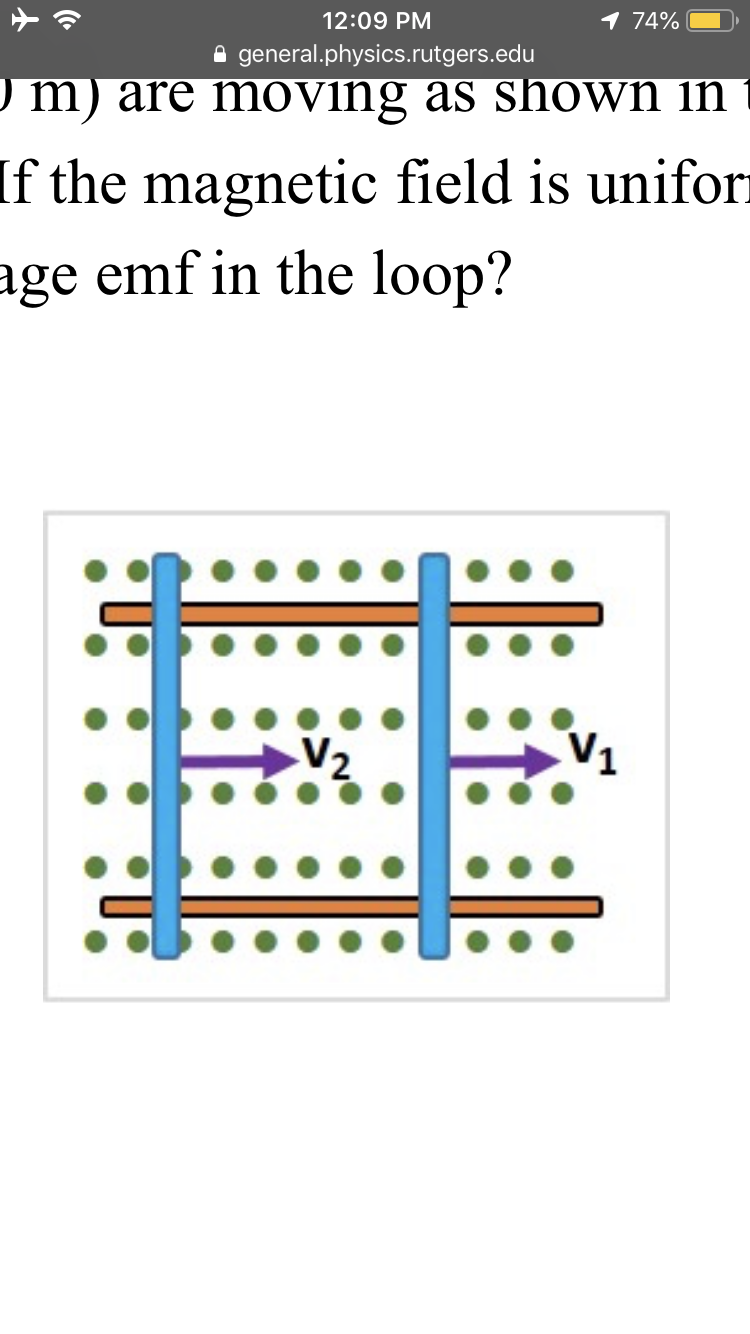# 1 74%12:09 PMgeneral.physics.rutgers.edu) m) are moving as shown inIf the magnetic field is uniforage emf in the loop?

Question
48 views

Two rods of equal lengths (1.40 m) are moving as shown in the figure. The speed v1 = 2.00 m/s and the speed v2 = 1.10 m/s. If the magnetic field is uniform with a magnitude of 0.45 T, what is the magnitude of the average emf in the loop?help_outlineImage Transcriptionclose1 74% 12:09 PM general.physics.rutgers.edu ) m) are moving as shown in If the magnetic field is unifor age emf in the loop? fullscreen
check_circle

Step 1

Given

Length of the rods L = 1.40 m

Speed of the rods

v1 = 2.00 m/s

V2 = 1.10 m/s

Magnetic field B = ...

### Want to see the full answer?

See Solution

#### Want to see this answer and more?

Solutions are written by subject experts who are available 24/7. Questions are typically answered within 1 hour.*

See Solution
*Response times may vary by subject and question.
Tagged in

### Physics